# Calculate Number of Months Between Dates in Excel & Google Sheets

This tutorial will demonstrate how to calculate the number of months between two dates in Excel & Google Sheets.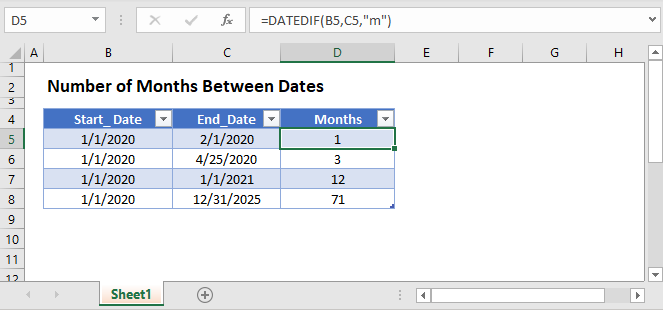## Number of Months Between Dates

To calculate the number of months between dates you can use the DATEDIF Function:

``=DATEDIF(B3,C3,"m")``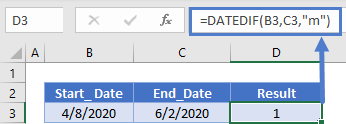Notice that the DATEDIF Function only counts fully completed months. It does not return a fractional value.

You can use the “md” input to calculate the number of days from the partially completed month:

``=DATEDIF(B3,C3,"md")``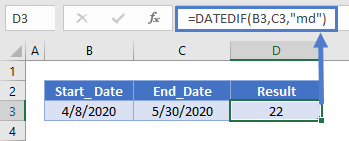and you can join the results together in a string of text to display the months and days difference between the dates:

``=DATEDIF(B3,C3,"m")&" Months and "&DATEDIF(B3,C3,"Md")&" Days "``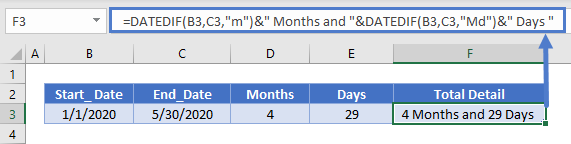### Fractional Month

Using the calculations above, you can calculate an approximate fractional month value by dividing the “md” DATEDIF function result by 30 and adding it to the first function result.

``=DATEDIF(B3,C3,"m")+ROUND((DATEDIF(B3,C3,"m")/30),2)&" Months"``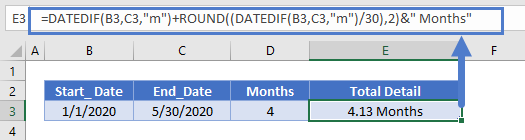## Number of Months Between Dates in Google Sheets

All of the above examples work exactly the same in Google Sheets as in Excel.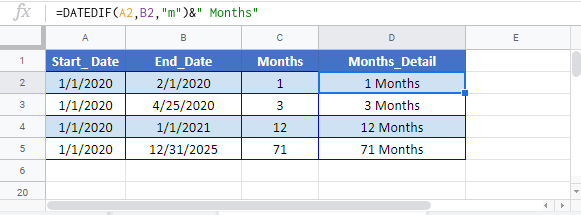### Excel Practice Worksheet

Practice Excel functions and formulas with our 100% free practice worksheets!